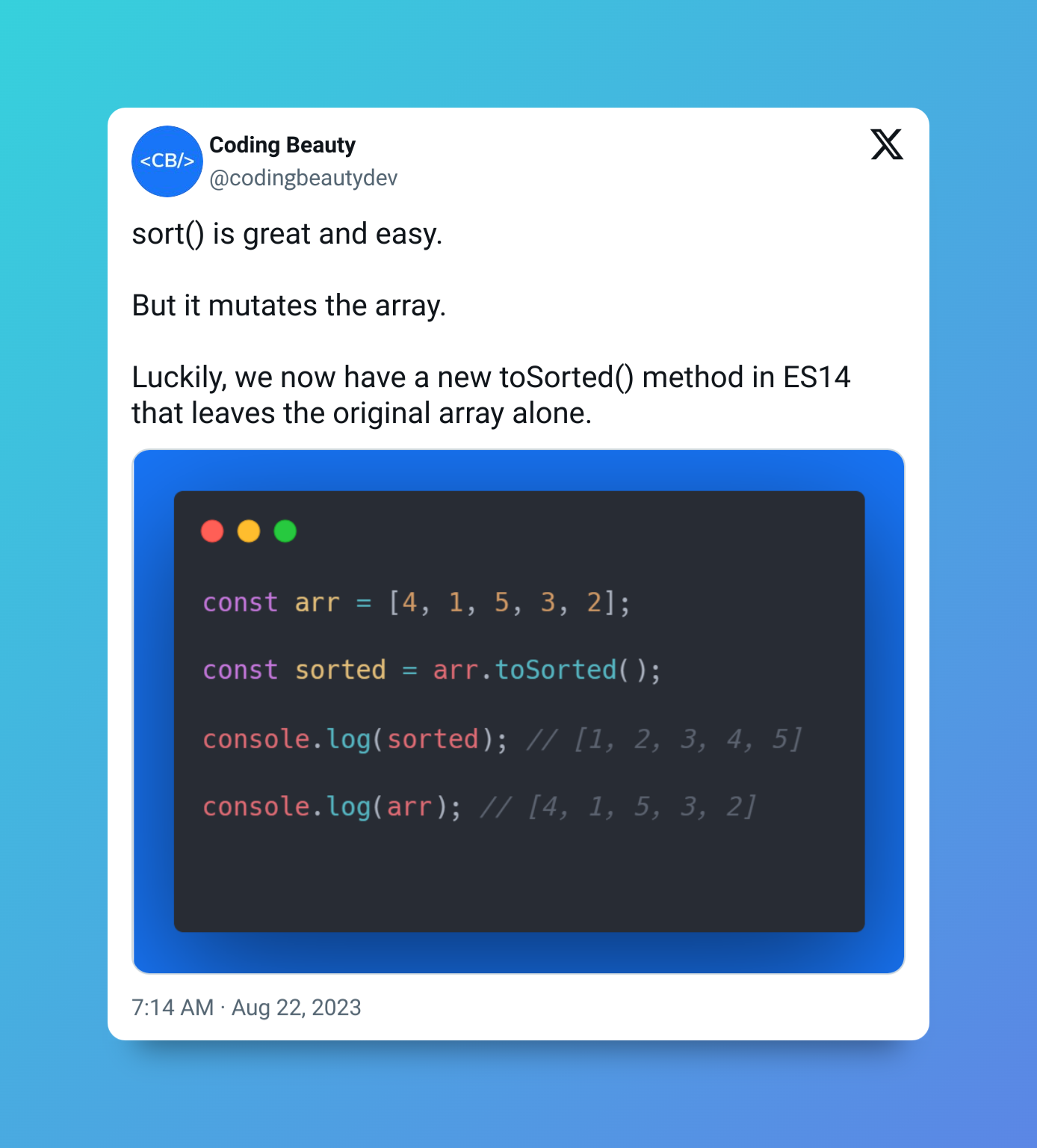# How to quickly get the union of two Sets in JavaScript

To get the union of two Sets in JavaScript, convert the sets to arrays and merge them, then create a `Set` from the merged array, i.e., `new Set([...set1, ...set2])`.

For example:

JavaScript
``````function getUnion(set1, set2) {
return new Set([...arr1, ...arr2]);
}

const set1 = new Set([1, 2, 3, 4]);
const set2 = new Set([3, 4, 5, 6]);

const union = getUnion(set1, set2);

console.log(union); // Set(6) { 1, 2, 3, 4, 5, 6 }``````## `Set()` constructor

The `Set()` constructor creates a new `Set` object from an iterable like an array. As a `Set` can only contain unique values, it removes any possible duplicates that may be in the array it receives.

JavaScript
``````const set = new Set([1, 1, 2, 3, 3, 4]);
console.log(set); // Set(4) [1, 2, 3, 4]``````

## Spread syntax (`...`)

The array we pass to `Set()` is a merge of two arrays that represent the two `Set`s. The spread syntax (`...`) unpacks the value of each `Set` into the array.

JavaScript
``````const array1 = [10, 20, 30];
const array2 = [40, 50, 60];

const mergedArray = [...array1, ...array2];

console.log(mergedArray);``````

## Use `Array concat()` to merge arrays

If you like, you can use the `Array concat()` method to join the arrays. Here the spread syntax only converts the `Set`s to arrays.

JavaScript
``````function getUnion(set1, set2) {
// Using Array concat() in place of spread syntax
return new Set([...set1].concat([...set2]));
}

const set1 = new Set([1, 2, 3, 4]);
const set2 = new Set([3, 4, 5, 6]);

const union = getUnion(set1, set2);

console.log(union); // Set(6) { 1, 2, 3, 4, 5, 6 }``````

## Use `Array from()` to convert `Set` to array

You can use the `Array from()` method in place of the spread syntax (`...`) to convert the `Set`s to arrays before merging them:

JavaScript
``````function getUnion(set1, set2) {
// Using Array concat() in place of spread syntax
return new Set(Array.from(set1).concat(Array.from(arr2));
}

const set1 = new Set([1, 2, 3, 4]);
const set2 = new Set([3, 4, 5, 6]);

const union = getUnion(set1, set2);

console.log(union); // Set(6) { 1, 2, 3, 4, 5, 6 }``````

We can use a similar approach to easily get the union of two arrays in JavaScript.

## Get union of two arrays in JavaScript

To get the union of two arrays in JavaScript, merge the arrays, create a new `Set` object from the merged result to remove the duplicates, then convert it back to an array, i.e., `Array.from(new Set([...arr1, ...arr2]))`.

For example:

JavaScript
``````function getUnion(arr1, arr2) {
return Array.from(new Set([...arr1, ...arr2]));
}

const array1 = [1, 2, 3, 4, 5];
const array2 = [4, 5, 6, 7, 8];

const union = getUnion(array1, array2); // [1, 2, 3, 4, 5, 6, 7, 8]
console.log(union);``````

## Key takeaways

• To get the union of two `Set`s in JavaScript, convert the `Set`s to arrays, merge them, and convert the merged array back to a `Set` object, i.e., `new Set([...set1, ...set2])`.
• You can get the union of two arrays in JavaScript in a similar way, i.e., `[...new Set([...arr1, ...arr2])]`.

## Every Crazy Thing JavaScript Does

A captivating guide to the subtle caveats and lesser-known parts of JavaScript.